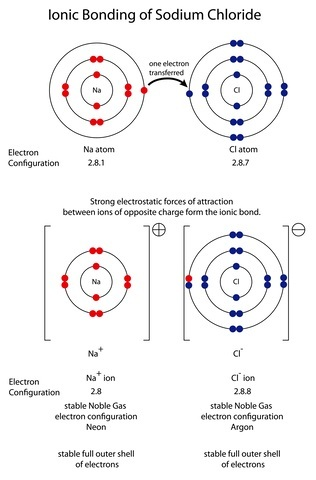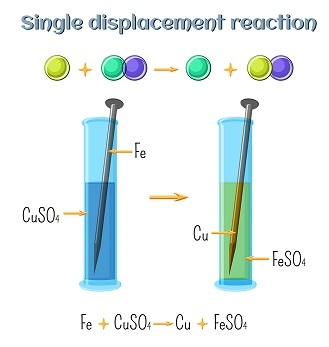# Types of Reactions Experiment

## Introduction

Different types of reactions experiment may be seen in the particles when these are passing through chemical reactions. Internal as well as external changes can happen in this reaction or experiment. In these types of reactions, the primary substances does not exerts with any changes in physical properties like, the changes of freezing point or evaporation temperature etc.

## What is a Reaction Experiment?

Reaction experiment is an integral part of the chemical field and different types of reactions are arranged to form new molecules or change the physical as well as chemical substances.

The excitement of the chemical field is completely dependent on the power of reactivity of the molecules.

Various kinds of experiments are seen and for those reactions, the processes of the experiments are different as well. Combination and decomposition are the most useful and applied experiments in the chemical field. Single displacement reaction experiment along with combustion is other types of reaction are very significant in the chemical field.

## Combination Reaction

This reaction requires two different molecules that react and then form a new compound. This kind of reaction is also called a synthesis reaction because in a single reaction, rearrangement along with combination of double or more particles happen for the formation of the product. A simple example of this type of reaction is presented as follows −

$$\mathrm{X=Y = XY}$$Figure 1: Formation of NaCl

The real example of this type of reaction is the formation of NaCl as in this reaction the sodium and chloride are reacted to form sodium chloride. This reaction is presented as

$$\mathrm{2\:Na (s) + Cl_2 (g)\:\rightarrow\:2NaCl (s)}$$

Another experiment of this type of reaction is seen with $\mathrm{O_2}$ and in this reaction; a metallic or non-metallic body is reacted with oxygen for forming oxides. This reaction is denoted as −

$$\mathrm{2\:Mg (S) + O_2 (g)\:\rightarrow\:2MgO (s)}$$

## Decomposition Reaction

This particular type of reaction is a little different from the previous reaction. In this reaction, a complex molecule breaks into a simple molecule. The decomposition experiment requires a specific energy source which is essential for breaking the present bonds of the molecules. It denotes the breaking down of the compounds into a simple compound or molecule. An example of this type of reaction is as follows −

$$\mathrm{XY = X+Y}$$

It mainly takes place in the existence of different natural sources like ,light, heat, as well as electricity. A simple decomposition reaction is arranged when a binary molecule is directly composed for forming a new product. An example is as follows −

$$\mathrm{2\:HgO (s)\:\rightarrow\:2\:Hg (l) + O_2 (g)}$$

It displays that the reactant can easily be transformed into a particular form. The form can be element or compound. Through it process, $\mathrm{CaCO_3}$ is decomposed with some molecules like calcium oxide along with carbon dioxide. Bases as well as alkalis easily are decomposed after heating. The reaction is denoted as

$$\mathrm{2\:NaOH (s) + Na_2O (s) + H_2O (g)}$$

## Single Displacement Reaction Experiment

This is a complex type of compound and in this reaction; a single element of reactant is replaced with an almost similar type of element of various reactants. It is also called a single replacement reaction experiment because it relies upon the chemical reactivity of the molecules. High reactivity elements are mainly replaced with the lower reactive elements in this reaction. It is denoted as −

$$\mathrm{X+YZ = XY+Z}$$Figure 2: Reaction of metallic iron displaces copper ion

An example of this experiment is seen between magnesium and copper and in this reaction magnesium mainly replaces copper because the reactivity power of magnesium is higher than copper. It reaction can be presented as −

$$\mathrm{Mg\:(s) + Cu(NO_3)_2 (aq)\:\rightarrow\: Mg(NO_3)_2\:(aq) + Cu\:(s)}$$

Another example of this type of reaction is as follows −

$$\mathrm{Fe(s) + CuSO_4(aq)\:\rightarrow\:FeSO_4(aq) + Cu(s)}$$

## Combustion Reaction

This type of reaction is a particular chemical experiment where the reactants are involved in the reaction with the help of oxygen and then release a certain amount of energy as well as heat. This reaction mainly occurs in the existence of oxygen and two different types of reaction can be seen such as incomplete and complete combustion.

It displays that the organic molecules undergo a complete combustion producing $\mathrm{CO_2}$ and $\mathrm{H_2O}$ in the gas form and releasing energy. The reaction is presented as −

$$\mathrm{C_3H_8 (g) +5O_2 (g)\:\rightarrow\:3CO_2 (g) + 4H_2O (g)}$$

It shows that the hydrocarbons are applied in this reaction for the production of energy. This type of reaction refers to as an exothermic reaction.

## Conclusion

The reactions not only are seen in the laboratory but in the surrounding of environment also. The duration of all reactions or experiments is not the same. The reaction rate of the different molecules is changed after adding a catalyst. Any kind of physical change is not usually seen in the reaction.

## FAQs

Q1. What is called a double displacement reaction?

Ans. The chemical reaction of this kind has positive and negative ions that exchange the position for forming a new compound. It mainly takes place in the iconic compounds and this reaction is presented as

$$\mathrm{AB + CD\:\rightarrow\:AD + CB}$$

Q2. What is the difference between single and double displacement reactions?

Ans. Only one compound is replaced by the other two reactants that rely upon reactivity in the single displacement reactions. The double displacement reactions guide to a reaction where reactant cations are exchanged as well as the anion too.

Q3. What is called the reactivity series of metals?

Ans. The reactivity series refers to a series where metals are organised for decreasing order of reactivity. Hydrogen is the only non-metallic element of this series for comparison purpose.

Updated on: 17-Apr-2023

162 Views